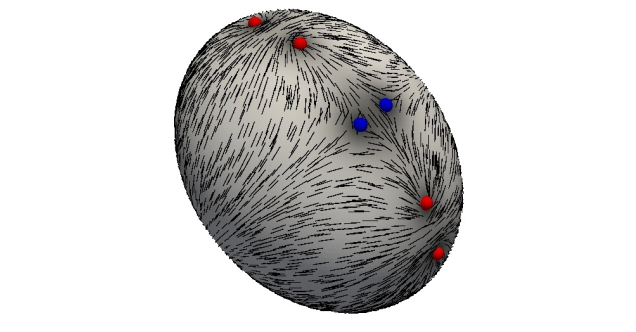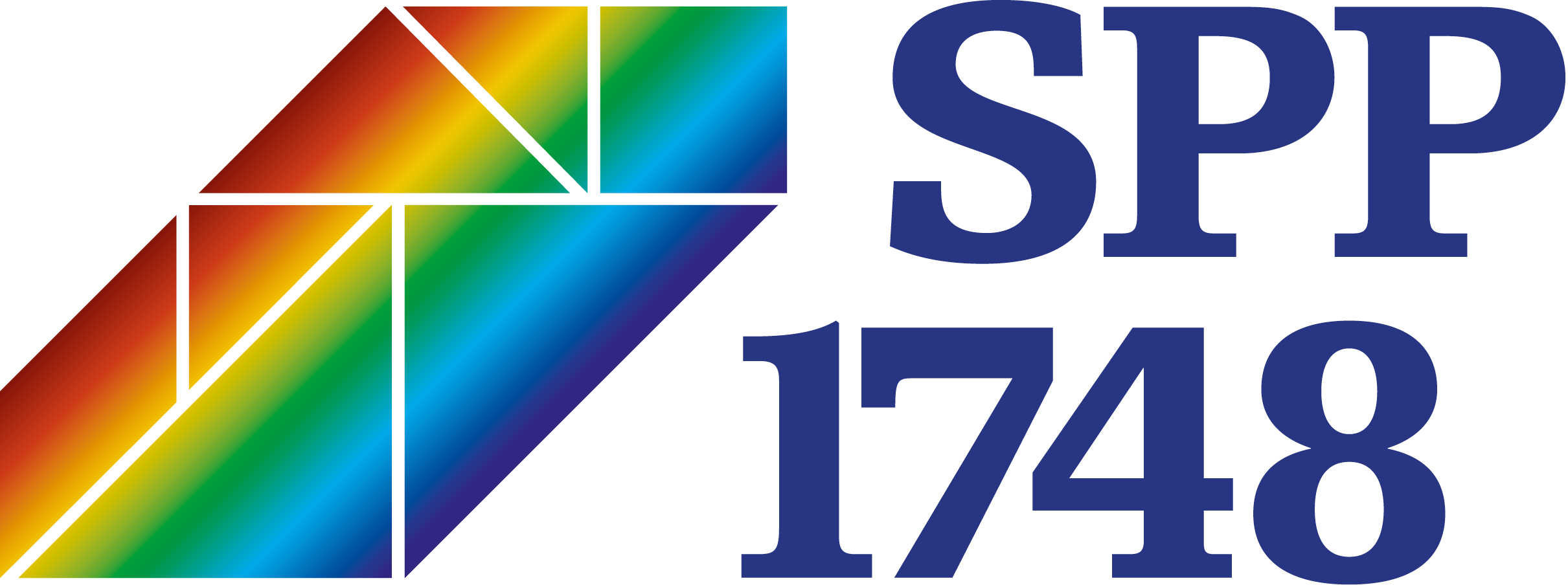# Funded projectsModeling and simulation of thin-sheet folding DFG prirority programme SPP 2256 Varational Methods for Predicting Complex Phenomena in Engineering Structures and Materials Funding period: 2020-2023

Description. Nonlinear bending theories for thin elastic objects have recently attracted considerable attention within applied mathematics. One reason for this is the seminal work by Friesecke, James and Müller on the rigorous justification of Kirchhoff's bending theory that inspired mathematical theories for describing multilayer materials, for predicting the occurrence of wrinkling phenomena, and for the precise modelling of twist effects in rods and ribbons. Another reason is the increasing interest in developing nanotechnological applications where slender objects undergo externally stimulated large deformations at low energies. Examples include the controlled fabrication of nanotubes, the imitation of flapping mechanisms in biology, and the improvement of aneurysma coiling methods in medical applications. An important and challenging aspect of nonlinear bending behaviour that has not been rigorously studied in the literature concerns the folding of thin elastic sheets along a prescribed arc along which the material is softened. Related mechanical effects are used in the construction of cardboxes and origami structures, fast folding effects in carnivorous plants, and the production of particular shapes of thin metal sheets. Curvature relations and boundary data determine whether folded isometries exist or whether wrinkling effects may occur. The proposed research project aims at the rigorous derivation of suitable lower dimensional models, the identification of analytical properties such as stability of arc perturbations, and the development of reliable and efficient numerical methods for the prediction of practically relevant model situations.Bending plates of nematic liquid crystal elastomers DFG research unit FOR 3013 Vector- and Tensor-Valued Surface PDEs Funding period: 2020-2023

Description. Programmable actuators are devices made of elastic materials whose shape can be controlled via external stimulation. Because of a wide range of technological applications particularly at small scales, they have received considerable attention in engineering, physics, and applied mathematics. A precise understanding of their behavior is crucial for their design and reliable use. This requires effective mathematical models and accurate numerical simulation methods. The proposed project focusses on the class of nematic thin films which are thin sheets of nematic liquid crystal elastomers. Starting from a coupled model using the frameworks of 3D hyperelasticity, and liquid crystal models (e.g., Oseen-Frank), different effective two-dimensional plate models will be derived that determine a possibly large deformation of the elastic thin film in connection with a corresponding orientation field describing the arrangement of the liquid crystals. Characteristic for the models are the occurrence of curvature quantities, constraints that enforce ruled surfaces, vector fields of unit or bounded length, and nonlinearities that define the coupling between the two quantities of interest. These special features require the use and development of appropriate numerical methods in order to simulate relevant effects within efficient implementations and to make reliable predictions for the development of new nanotools. A mathematically rigorous connection between the three-dimensional and the dimensionally reduced nonlinear plate bending model will be established via the concept of Gamma-convergence, a thorough convergence analysis for the proposed finite element discretization will be carried out avoiding unjustified regularity assumptions, and efficient iterative solution strategies will be investigated theoretically and experimentally.Approximation of non-smooth optimal convex shapes with applications in optimal insulation and minimal resistance DFG priority programme SPP 1962 Non-smooth and Complementarity-based Distributed Parameter Systems: Simulation and Hierarchical Optimization Funding period: 2020 - 2023

Description. Devising and analyzing numerical methods for shape optimization problems typically requires the restriction of the class of admissible shapes. In this project we aim at investigating the discretization and iterative solution of shape optimization problems with convexity constraint. This constraint leads to unexpected mathematical difficulties and phenomena. First, appropriate discrete notions of convexity are required to prevent locking effects of numerical methods, and second, optimal convex shapes are typically nonsmooth which necessitates a careful convergence analysis. Related applications involve constraints defined by partial differential equations and range from modelsfor optimal insulation with breaks of symmetry, and the design of bodies with low flow resistance or maximal torsion stiffness, to the determination of special shapes such as bodies of constant width in geometry. The goal of the project is to develop and analyze numerical methods for the reliable and efficient computation of optimal convex shapes and to identify optimal shapes in scientific and geometric applications. Particular aspects are the development of discrete notions of convexity, appropriate representations of shape derivatives, identification of mesh regularity and convexity preserving diffeomorphisms and compactness properties of discrete convex sets.Reliability of efficient approximation schemes for material discontinuities described by functions of bounded variation DFG priority programme SPP 1748 Reliable Simulation Techniques in Solid Mechanics. Development of Non-standard Discretization Methods, Mechanical and Mathematical Analysis Funding period: 2018 - 2021

Description. Spaces of functions of bounded variations provide an attractive framework to describe material discontinuities such as damage and fracture. Recently, suitable notions of solution and general existence theories for corresponding evolutionary model problems have been established and numerical methods for discretizing and iteratively solving variational problems involving the total variation norm have been developed and analyzed. In the first funding period of the project, abstract existence results for coupled rate-dependent/rate-independent systems, delamination processes in visco-elastodynamics, and phasefield descriptions of damage evolutions have been investigated analytically. Algorithmic contributions have been made to the iterative solution of model problems on functions of bounded variation, adaptive approximation of discontinuous functions based on fully computable a posteriori error estimates, and the convergent finite element simulation of a BV-regularized damage model. Within a second funding period these results will be combined, refined, and extended to complex models describing damage and fracture evolution. The envisaged models capture material discontinuities in BV either directly or as a scaling limit. The planned research includes the derivation of a~priori and a posteriori error estimates, the construction of adaptive approximation schemes intertwined with results based on existence theory and Gamma-convergence, and their implementation and application to specific benchmark problems in mechanics. Particular attention will be paid to the reliability of efficient methods, e.g., convergence of suitable time-stepping and adaptive approximation schemes based on variational techniques.Approximation of Monge-Kantorovich problems DFG priority programme SPP 1962 Non-smooth and Complementarity-based Distributed Parameter Systems: Simulation and Hierarchical Optimization Funding period: 2016 - 2019

Description. Optimal transportation of substances, goods, or information is a classical mathematical problem with applications in economics, meteorology, and computer science. It can be formulated as a high-dimensional linear program whose direct solution is difficult. More efficient approaches are based on equivalent continuous formulations via partial differential equations or variational problems. These are nonlinear and nondifferentiable mathematical problems that require a suitable discretization and iterative solution. The project focuses on the developement and numerical analysis of finite elemet discretizations, the automatic efficient mesh refinement based on rigorous a posteriori error estimates, and the fast iterative solution. Special emphasis is on the avoidance of regularizations and use of unjustified smoothness assumptions on solutions.Finite element approximation of functions of bounded variation and application to models of damage, fracture and plasticity DFG priority programme SPP 1748 Reliable Simulation Techniques in Solid Mechanics. Development of Non-standard Discretization Methods, Mechanical and Mathematical Analysis Funding period: 2014 - 2017

Description. The aim of this project is to establish reliable and efficient numerical methods for models of solids with spatial discontinuities caused by the evolution of dissipative processes such as plasticification, damage or fracture. In particular, the project focuses on such prototypical models that use the class of BV-functions to mathematically describe the discontinuities, that are guaranteed to converge to a solution of the infnite-dimensional model and for which iterative solution methods can be constructed. Emphasis is on unregularized numerical approaches that lead to sharp approximations of discontinuities on coarse grids and rigorous convergence proofs. The main objectives of the research project are the development, analysis, and implementation of finite element methods for model problems describing discontinuities in BV. This includes the derivation of a priori and a posteriori error estimates as well as the construction of adaptive and extended approximation methods for BV-prototype models such as the Rudin-Osher-Fatemi and the Mumford-Shah model. The techniques will be transferred to analytically justified and closely related models for the description of rate-independent inelastic processes, in particular perfect plasticity, damage and fracture. The methods and results will be applied and transferred to particular model scenarios and benchmark problems in mechanics.Reliable approximation of singularities in geometric partial differential equations Collaborative Research Center SFB 611 Singular Phenomena and Scaling in Mathematical Models Funding period: 2009 - 2012

Description. The project investigates the development and analysis of numerical methods for the approximation of geometric partial differential equations. Focus is on the reliability of algorithms for weak solutions which may exhibit singularities and blowup phenomena in finite time. Applications include the simulation of switching processes in micromagnetics, surface registration in image processing, and the prediction of patterns of lipids and shapes of biological membranes.Simulation of phase field models and geometric evolution problems Research Center Matheon Mathematics in Key Technologies Funding period: 2005 - 2008

Description. Allen-Cahn equations, which are the simplest case of Phase field models, occur in the mathematical modeling of various physical processes and applications in several key technologies such as phase transitions in binary alloys and superconducting materials. They are often employed for the approximation of nonlinear geometric evolution problems which arise in liquid crystal theory, micromagnetics, and color image denoising. Solutions depend sensitively on a small parameter. Owing to the limited practical regularity of these solutions, numerical schemes have to be carefully chosen in order to yield reliable approximations. In fact, classical error estimates for the numerical approximation depend exponentially on the small parameter and are therefore of limited practical use. It has only recently been shown that a robust error estimation is possible in certain situations. The proposed schemes employ or compute spectral estimates for the linearized partial differential equation about the exact or approximate solution and thereby measure the stability of a solution. In the Project C16, adaptive numerical schemes will be designed for Cahn-Hilliard and Cahn-Larche equations which allow robust error estimation. In particular, a posteriori error estimates will be derived which are the basis for local mesh-refinement and coarsening and thereby yield efficient approximation schemes. Convergence of adaptive approximation schemes will be investigated and numerical simulations will be carried out for a mathematical model in the production of lead-free electric joints in microeletronic devices. A rigorous mathematical analysis of projection schemes and fully implicit discretization methods for the approximation of geometric evolution problems will be carried out. Finite time blow-up of solutions for Landau-Lifshitz-Gilbert equations will be studied numerically and theoretically.